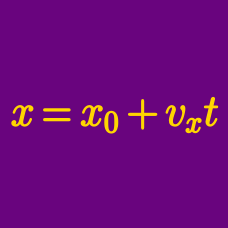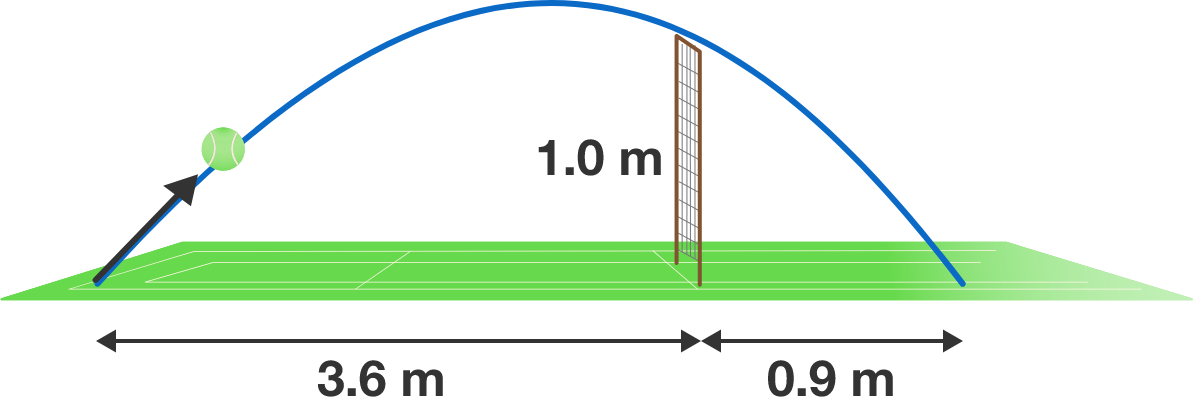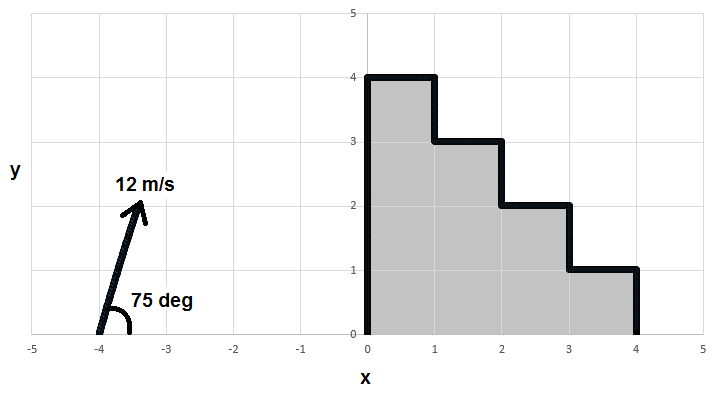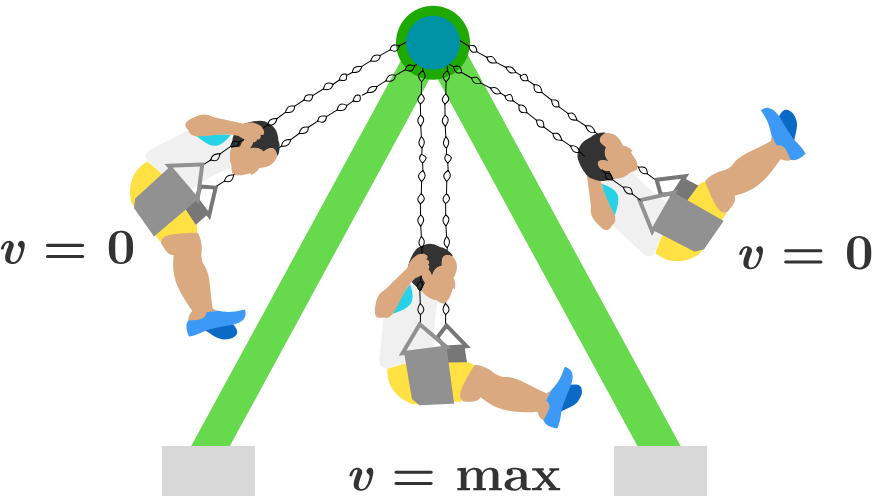Classical Mechanics

# 2D Kinematics: Level 2-4 Challenges

A stone is thrown from the surface of Earth, where the acceleration due to gravity is $9.8 \text{ m/s}^2.$ The stone travels along a parabolic trajectory.

If the same stone is thrown exactly the same way from the surface of Mars, where the acceleration due to gravity is $3.7 \text{ m/s}^2,$ then the Martian trajectory is $\text{\_\_\_\_\_\_\_\_\_\_}$ the trajectory on Earth,

A "drop shot" in tennis is a shot that barely passes the net and touches the other side of the court close to the net. This makes it difficult for the opponent to reach the ball if the opponent starts running for the ball from the baseline.Suppose you hit a drop shot $3.6\text{ m}$ from your side of the court, making the ball leave your racket very close to the ground. The ball then clears the net at a height of $1\text{ m}$ and touches the ground $0.9\text{ m}$ from the net on the opponent's side, as shown in the diagram.

How long (in seconds) is the ball in the air before hitting the ground after you hit the drop shot, to 2 decimal places?


Details and Assumptions:

• Neglect air resistance.
• The plane containing the trajectory of the ball is perpendicular to the net.
• Use $g = 10 \text{ m/s}^2$.

From the origin of a Cartesian coordinate system, a particle of mass $m$ is thrown with an initial speed $v_{0}$ at an angle of $\theta=\frac{\pi}{3}$ from the horizontal x-axis. The air friction $f$ acts on the particle such that $\vec{f} = - b\vec{v},$ where $b$ is a positive constant and $\vec{v}$ is the velocity of the particle. If the x cordinate where the particle will only have vertical speed can be writen as $x=\dfrac{\alpha\cdot mv_{0}}{\beta \cdot b}.$ Find the value of $\alpha+\beta.$

The gravity acts in the vertically downward direction as $\vec{g}=-g\hat{y}$.

For more problems, visit my set.A projectile is launched from an initial position $(x,y) = (-\SI{4}{\meter},\SI{0}{\meter})$. The projectile's initial speed is $12\text{ m/s}$ and it is launched at a $\SI{75}{\degree}$ angle with respect to the $x$-axis. There is a staircase with 4 steps, as pictured above. The top step begins at $(x,y) = (\SI{0}{\meter},\SI{4}{\meter})$ meters and the bottom step ends at $(x,y) = (\SI{4}{\meter},\SI{0}{\meter})$. Each step is $\SI{1}{\meter}$ wide and $\SI{1}{\meter}$ deep. The projectile flies over part of the staircase and lands on one of the steps. What is the $x$ coordinate of the landing point in meters?

Details and Assumptions

• The gravitational acceleration is in the $-y$ direction, with a magnitude of $\SI{9.8}{\meter\per\second\squared}$.A little boy swings back and forth on the playground. At the highest point in his swing his speed is zero, and at his lowest point his speed is greatest.

Where in the trajectory will his acceleration be zero?

×